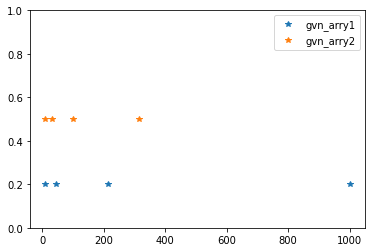# Logspace python – Python NumPy logspace() Function

NumPy logspace() Function:

Logspace python: The logspace() method in the NumPy module returns numbers that are evenly spaced on a log scale.
The values are generated with the number of samples given in the range [base ** start, base ** stop]. The interval’s endpoint can be excluded if desired(optionally).

Syntax:

numpy.logspace(start, stop, num=50, endpoint=True, base=10.0, dtype=None)

Parameters

start: This is required. It specifies the starting value of the sequence. base ** start is the starting value.

stop: This is required. Unless endpoint is False, specify the sequence’s end value (end value is base ** end). In such a scenario, num + 1 values are spaced across the interval in log-space, with all but the last (a sequence of length num) being returned.

num: This is optional. It represents the number of samples to generate. The default value is 50. It must not be negative(non-negative).

endpoint: This is optional. It represents the boolean value. If True, stop is the last sample. Otherwise, it is not included. True is the default.

base: This is optional. It represents the base of the log space. The element step size in ln(samples) / ln(base) (or log base(samples)) is uniform. The default value is 10.0.

dtype: This is optional. It represents the output array’s type. If dtype is not specified, the data type is inferred from the other input arguments.

Return Value:

Returns an array containing elements within the given range.

## NumPy logspace() Function in Python

Example1

Approach:

• Import numpy module using the import keyword
• Pass some random start, stop values and number of samples(num)
as arguments to the logspace() function and store it in a variable.
• Here it creates the given number of sample points
• Print the given first array
• Pass some random start, stop values, number of samples(num) and endpoint=False
as arguments to the logspace() function and store it in another variable.
• Here, When endpoint=False, (num+1) samples are generated, and samples
without the last one are returned.
• Print the given second array
• Similarly, do the same by adding some random base argument.
• Store it in another variable
• Print the given third array
• The Exit of the Program.

Below is the implementation:

# Import numpy module using the import keyword
import numpy as np

# Pass some random start, stop values and number of samples(num)
# as arguments to the logspace() function and store it in a variable.
# Here it creates the given number of sample points
gvn_arry1 = np.logspace(1, 3, num=4)
# Print the given first array
print("The given first array:", gvn_arry1)

# Pass some random start, stop values, number of samples(num) and endpoint=False
# as arguments to the logspace() function and store it in another variable.
# Here, When endpoint=False, (num+1) samples are generated, and samples
# without the last one are returned.
gvn_arry2 = np.logspace(1, 3, num=4, endpoint=False)
# Print the given second array
print("The given second array:", gvn_arry2)

# Similarly, do the same by adding some random base argument.
# Store it in another variable
gvn_arry3 = np.logspace(1, 3, num=4, base=2.0)
# Print the given third array
print("The given third array:", gvn_arry3)

Output:

The given first array: [ 10.  46.41588834  215.443469  1000. ]
The given second array: [ 10.  31.6227766  100.  316.22776602]
The given third array: [2.  3.1748021  5.0396842  8. ]

Example2

Numpy logspace: Here, the arrays are constructed using the endpoint argument of True and False, and the result is visualized using the matplolib module.

Below is the implementation:

# Import numpy module using the import keyword
import numpy as np
# Import numpy module using the import keyword
import matplotlib.pyplot as plt
# Pass some random start, stop values, number of samples(num) and endpoint=True
# as arguments to the logspace() function and store it in a variable.
# Here it creates the given number of sample points
gvn_arry1 = np.logspace(1, 3, num=4, endpoint=True)
# Print the given first array
print("The given first array:", gvn_arry1)

# Pass some random start, stop values, number of samples(num) and endpoint=False
# as arguments to the logspace() function and store it in another variable.
# Here, When endpoint=False, (num+1) samples are generated, and samples
# without the last one are returned.
gvn_arry2 = np.logspace(1, 3, num=4, endpoint=False)
# Print the given second array
print("The given second array:", gvn_arry2)
k = np.zeros(4)

# Plot the graph for the above given two arrays using the plot() function
# of the matplotlib module
plt.plot(gvn_arry1, k+0.2, '*')
plt.plot(gvn_arry2, k+0.5, '*')
plt.ylim([0, 1])
plt.legend(labels = ('gvn_arry1', 'gvn_arry2'))
plt.show()

Output:

The given first array: [  10.           46.41588834  215.443469   1000.        ]
The given second array: [ 10.          31.6227766  100.         316.22776602]